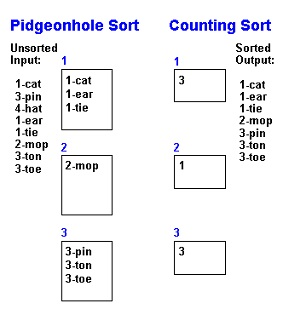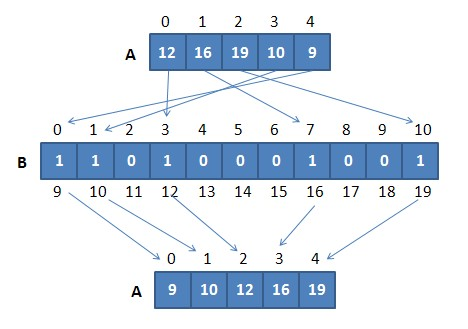# Pigeonhole Sort Basic Information

suggest change

Pigeonhole Sort is a sorting algorithm that is suitable for sorting lists of elements where the number of elements (n) and the number of possible key values (N) are approximately the same. It requires O(n + Range) time where n is number of elements in input array and ‘Range’ is number of possible values in array.

Working(Pseudo code) for Pigeonhole Sort:

1. Find minimum and maximum values in array. Let the minimum and maximum values be ‘min’ and ‘max’ respectively. Also find range as ‘max-min-1′.
2. Set up an array of initially empty “pigeonholes” the same size as of the range.
3. Visit each element of the array and then put each element in its pigeonhole. An element input[i] is put in hole at index input[i] – min.
4. Start the loop all over the pigeonhole array in order and put the elements from non- empty holes back into the original array.

Pigeonhole sort is similar to counting sort, so here is a comparison between Pigeonhole Sort and counting sort.Example of Pigeonhole Sort:Space Auxiliary: `O(n)`

Time Complexity: `O(n + N)`